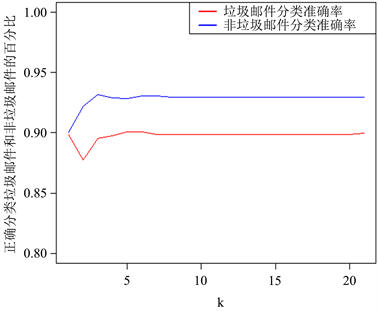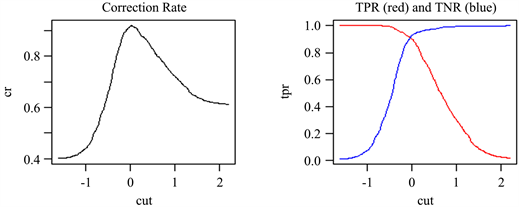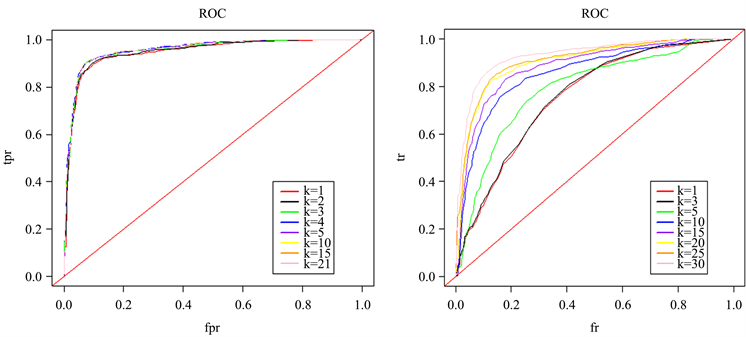﻿ 基于偏最小二乘回归的邮件分类问题研究

# 基于偏最小二乘回归的邮件分类问题研究Research on Mail Classification Problem Based on Partial Least Squares Regression

Abstract: This paper classifies emails based on the principal component regression (PCR) method of least squares, and further uses partial least squares regression (PLS) to identify and classify spam emails. The classification accuracy obtained by PLS and PCR was compared and analyzed. Then the trend of the percentage of classification accuracy of PLS with the classification cut-off point is examined, and the ROC curve corresponding to different k values (number of principal components) under the two methods is obtained. Finally, this article analyzes the accuracy and stability of PLS and PCR methods to identify and classify spam.

1. 引言

2. 研究问题

${\mathrm{min}}_{\beta \in {R}^{p}}{‖X\beta -y‖}_{2}$ (1)

${\mathrm{min}}_{\alpha \in {R}^{p}}{‖X{\Gamma }_{K}\alpha -y‖}_{2}$ (2)

3. 研究方法

3.1. PCR

PCR主要使用了SVD分解，对于 $X=U\left(\begin{array}{c}D\\ 0\end{array}\right){V}^{T}$，令 ${\Gamma }_{k}=\left({V}_{1},\cdots ,{V}_{k}\right)$，即是我们要求的 ${\Gamma }_{k}$，且 ${\Gamma }_{k}$

3.2. PLS

3.2.1. LGK双对角化的概念

$Z=P\left(\begin{array}{c}B\\ 0\end{array}\right){W}^{T}$ (3)

$B=\left(\begin{array}{ccccc}{b}_{1}& {a}_{1}& & & \\ & {b}_{2}& {a}_{2}& & \\ & & \ddots & \ddots & \\ & & & {b}_{p}& {a}_{p}\\ & & & & {a}_{p+1}\end{array}\right)$ (4)

${\Gamma }_{k}$ 为矩阵 $\left({w}_{2},{w}_{3},\cdots ,{w}_{k}\right)$ 删去第一行。

3.2.2. LGK双对角化的性质

${p}_{i}:n×1$ 为矩阵P的第i列， ${w}_{i}:\left(p+1\right)×1$ 为矩阵W的第i列，LGK双对角化性质如下：

· 令公式(3)左右同时左乘 ${P}^{T}$，可以得到：

${\left({p}_{1}:{p}_{2}:\cdots :{p}_{p+1}\right)}^{T}Z=B{W}^{T}=\left(\begin{array}{ccccc}{b}_{1}& {a}_{1}& & & \\ & {b}_{2}& {a}_{2}& & \\ & & \ddots & \ddots & \\ & & & {b}_{p}& {a}_{p}\\ & & & & {a}_{p+1}\end{array}\right)\left(\begin{array}{c}{w}_{1}^{T}\\ {w}_{2}^{T}\\ ⋮\\ {w}_{p+1}^{T}\end{array}\right)$ (5)

· 令公式(3)左右同时右乘W，可以得到：

${\left({p}_{1}:{p}_{2}:\cdots :{p}_{p+1}\right)}^{T}Z=B{W}^{T}=\left(\begin{array}{ccccc}{b}_{1}& {a}_{1}& & & \\ & {b}_{2}& {a}_{2}& & \\ & & \ddots & \ddots & \\ & & & {b}_{p}& {a}_{p}\\ & & & & {a}_{p+1}\end{array}\right)\left(\begin{array}{c}{w}_{1}^{T}\\ {w}_{2}^{T}\\ ⋮\\ {w}_{p+1}^{T}\end{array}\right)$ (6)

${p}_{i}=\frac{1}{{b}_{i}}\left(Z{w}_{i}-{a}_{i-1}{p}_{i-1}\right),\text{\hspace{0.17em}}\text{\hspace{0.17em}}i=2,\dots ,p,\left(p+1\right)$ (7)

· LGK的 矩阵第一列有 $p=\frac{y}{{b}_{1}}$，且W矩阵具有如下形式：，则 ${b}_{1}={‖y‖}_{2}$${p}_{1}=\frac{y}{{‖y‖}_{2}}$${w}_{1}=\left(1,0,0,\cdots ,0\right)$

LGK这三条性质为P、B、W矩阵的迭代算法提供了迭代初始值和迭代关系式。

4. 算法实现

4.1. P, W矩阵的迭代算法

${w}_{1}={\left(100\cdots 0\right)}^{T},\text{\hspace{0.17em}}\text{\hspace{0.17em}}{b}_{1}={‖y‖}_{2},\text{\hspace{0.17em}}\text{\hspace{0.17em}}{p}_{1}=\frac{y}{{‖y‖}_{2}}=\frac{b}{y}$

${a}_{1}={‖{z}^{T}{p}_{1}-{b}_{1}{w}_{1}‖}_{2}$

${w}_{2}=\frac{1}{{a}_{1}}\left({z}^{T}{p}_{1}-{b}_{1}{w}_{1}\right),\text{\hspace{0.17em}}\text{\hspace{0.17em}}{b}_{2}={‖z{w}_{2}-{a}_{1}{p}_{1}‖}_{2}$

${p}_{2}=\frac{1}{{b}_{2}}\left(z{w}_{2}-{a}_{1}{p}_{1}\right),\text{\hspace{0.17em}}\text{\hspace{0.17em}}{a}_{2}={‖{z}^{T}{p}_{2}-{b}_{2}{w}_{2}‖}_{2}$

${w}_{3}=\frac{1}{{a}_{2}}\left({z}^{T}{p}_{2}-{b}_{2}{w}_{2}\right),\text{\hspace{0.17em}}\text{\hspace{0.17em}}{b}_{3}={‖z{w}_{3}-{a}_{2}{p}_{2}‖}_{2}$

${p}_{3}=\frac{1}{{b}_{3}}\left(z{w}_{3}-{a}_{2}{p}_{2}\right),\text{\hspace{0.17em}}\text{\hspace{0.17em}}{a}_{3}={‖{z}^{T}{p}_{3}-{b}_{3}{w}_{3}‖}_{2}$

4.2. 邮件分类算法

Step 1：将训练集数据代入迭代算法中，通过迭代最终得到 ${\Gamma }_{k}$，此时回归模型为：

$y=X{\Gamma }_{k}\alpha +\epsilon$

Step 2：将 $X{\Gamma }_{k}$ 看作新的变量，然后利用最小二乘法估计计算新的线性模型系数 $\stackrel{^}{\alpha }$，计算结果为：

$\stackrel{^}{\alpha }={\left(\left(X{\Gamma }_{k}\right)TX{\Gamma }_{k}\right)}^{-1}{\left(X{\Gamma }_{k}\right)}^{T}y$，预测值为： $\stackrel{^}{y}=X{\Gamma }_{k}\stackrel{^}{\alpha }$

Step 3：利用测试集去代入预测模型，将得到的预测值与真实值比较，计算相应的分类准确率(真阳率、真阴率)，图示不同k值对应的分类准确率以及ROC曲线图，分析该方法的整体识别分类效果。

5. 结果展示与分析

5.1. 不同k值下分类准确率分析Table 1. Classification accuracy under different k values

5.2. 不同分类截点下分类准确率分析Figure 1. Accuracy rate change trend graphFigure 2. Classification accuracy percentage line chart

5.3. 不同k值对应的ROC曲线图

· ROC曲线能容易地查出任意界限值时对垃圾邮件的识别能力。

· 选择最佳的诊断界限值。ROC曲线越靠近左上角，实验的准确性就越高，最靠近左上角的ROC曲线的点是错误最少的最好阈值，其假阳性和假阴性的总数最少。

· 两种及以上不同诊断试验对疾病识别能力的比较。越靠近左上角的曲线识别的准确度越高。Figure 3. ROC graph

6. 结论

 李雪, 孙建平. 一种改进的偏最小二乘回归方法研究 [J]. 仪器仪表用户, 2017, 24(5): 16-19+28.

 Eldén, L. (2004) Partial Least-Squares vs. Lanczos Bidiagonalization-I: Analysis of a Projection Method for Multiple Regression. Computational Statistics and Data Analysis, 46, 11-31.
https://doi.org/10.1016/S0167-9473(03)00138-5

 赵晓丹, 徐燕. 垃圾邮件分类技术对比研究[J]. 信息网络安全, 2014(2): 75-80.

 毛雪莲. 多重共线性问题的偏最小二乘估计[J]. 科技视界, 2019(27): 152-153.

 陈龙, 梁意文, 谭成予. 基于自适应性分类器的垃圾邮件检测[J]. 计算机工程, 2018, 44(5): 194-200.

 李雨亭. 基于深度学习的垃圾邮件文本分类方法[D]: [硕士学位论文]. 太原: 中北大学, 2018.

 黄鹤, 荆晓远, 董西伟, 吴飞. 基于Skip-gram的CNNs文本邮件分类模型[J]. 计算机技术与发展, 2019, 29(6): 143-147.

 段同庆, 鲁瑞, 史新军, 刘红伟, 邓晓伟, 马骏. 偏最小二乘回归在探索PCI治疗冠心病患者预后影响因素中的应用[J]. 中国卫生统计, 2019, 36(6): 824-828.

 丁学利, 任鹏. 基于偏最小二乘回归的空气质量数据校准研究[J]. 廊坊师范学院学报(自然科学版), 2020, 20(1): 9-14.

 Keshav, K. (2021) Partial Least Square (PLS) Analysis. Resonance, 26, 429-442.
https://doi.org/10.1007/s12045-021-1140-1

 王琦, 吴钟扬, 黄陈蓉, 潘磊. 基于词嵌入与生成对抗网络的垃圾邮件分类算法[J]. 南京工程学院学报(自然科学版), 2018, 16(3): 20-27.

 吴小晴, 万国金, 李程文, 林梦思, 曹书强. 一种改进TF-IDF的中文邮件识别算法研究[J]. 现代电子技术, 2020, 43(12): 83-86.

 徐梦龙, 黄家旺. 朴素贝叶斯算法在垃圾邮件过滤方面的应用[J]. 网络安全技术与应用, 2018(7): 46-47.

Top